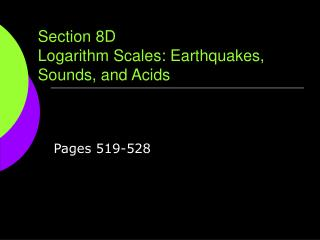DownloadDownload PresentationSection 8D Logarithm Scales: Earthquakes, Sounds, and Acids

# Section 8D Logarithm Scales: Earthquakes, Sounds, and Acids

Télécharger la présentation## Section 8D Logarithm Scales: Earthquakes, Sounds, and Acids

- - - - - - - - - - - - - - - - - - - - - - - - - - - E N D - - - - - - - - - - - - - - - - - - - - - - - - - - -
##### Presentation Transcript

1. Logarithmic Scales Earthquake strength isdescribed in magnitude. Loudness of sounds isdescribed in decibels. Acidity of solutions isdescribed by pH. Each of these measurement scales involves exponential growth. Successive numbers on the scale increase by the same relative amount. e.g. A liquid with pH 5 is ten times more acidic than one with pH 6.

2. Earthquake Magnitude Scale The magnitude scale for earthquakes is defined so that each magnitude represents about 32 times as much energy as the prior magnitude. The magnitude M is related to the released energy E by the following formulas: log10(E) =4.4 + 1.5M or E = (2.5 x 104) x 101.5M Energyis measured injoules. Magnitudeshaveno units.

3. ex1/521Calculate precisely how much more energy is released for each 1 magnitude on the earthquake scale (about 32 times more). Magnitude 1: E = (2.5 x 104) x 101.5(1) Magnitude 2: E = (2.5 x 104) x 101.5(2) Magnitude 3: E = (2.5 x 104) x 101.5(3) For each 1 magnitude, 101.5 = 31.623 times more energy is released.

4. ex2/521 How much energy was released by the magnitude 7.1 earthquake in San Francisco in 1989? by the magnitude 6.9 earthquake in Afghanistan in 1998? SF in 1989 with M=7.1: E = (2.5 x 104) x 101.5(7.1) = 1.11671E15 joules Afghanistan in 1998 with M=6.9: E = (2.5 x 104) x 101.5(6.9) = 5.5968E14 joules Since 1.11671E15/5.5968E14 = 1.99526, we say that: the SF quake was 1.99526 times more powerful than the Afghanistan quake.

5. Typical Sounds in Decibels decibels increase by 10 and intensity is multiplied by 10.

6. Decibel Scale for Sound Theloudness of a sound in decibels is defined by the following equivalent formulas:

7. More Practice 15/527 How many times as loud as the softest audible sound is the sound of busy street traffic? 17/527 What is the loudness, in decibels, of a sound 45 million times as loud as the softest audible sound? 19/527 How much louder (more intense) is a 35-dB sound than a 10-dB sound?

8. pH Scale for Acidity The pH is used by chemists to classify substances as neutral, acidic, or basic/alkaline. Pure Water is neutral and has a pH of 7.. Acids have a pH lower than 7. Bases have a pH higher than 7

9. The pH Scale The pH Scale is defined by the following equivalent formulas: pH = -log10[H+] or [H+] = 10-pH where [H+] is the hydrogen ion concentration in moles per liter.

10. Practice 27/527 What is the hydrogen ion concentration of a solution with pH 8.5? 29/527 What is the pH of a solution with a hydrogen ion concentration of 0.1 mole per liter? Is this solution an acid or base?

11. Homework Pages 526 - 527 # 12,14, 18,20, 28,30